# Olympiad Test: Direct And Inverse Proportions

## 20 Questions MCQ Test Mathematics (Maths) Class 8 | Olympiad Test: Direct And Inverse Proportions

Description
Attempt Olympiad Test: Direct And Inverse Proportions | 20 questions in 20 minutes | Mock test for Class 8 preparation | Free important questions MCQ to study Mathematics (Maths) Class 8 for Class 8 Exam | Download free PDF with solutions
QUESTION: 1

### Observe the given table.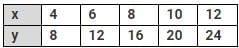What can be said about x and y?

Solution:

Clearly,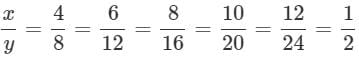∴ x and y are directly proportional as x/y is a constant.

QUESTION: 2

### A van covers 432 km with 36 litres of diesel. How much distance would it cover with 25 litres of diesel?

Solution:

In 36 litre of diesel a van cover 432 km

In 1 litre of diesel a van cover 432/36 =12 km

In 25 litre of diesel a van cover 12×25 = 300 km

QUESTION: 3

### A photograph of a type of bacteria enlarged 50000 times attains a length of 5 cm. What is the actual length of bacteria?

Solution:

Actual length of the bacteria is 5/50,000 = 0.0001 cm = 10−4 cm

QUESTION: 4

If the cost of 27 bags of paddy is Rs.9450, what is the cost of 36 bags of paddy?

Solution:
QUESTION: 5

The scale of a map is 1:3×107 Two cities are 5 cm apart on the map. Find the actual distance between them in kilometres.

Solution:
QUESTION: 6

A train is moving at a uniform speed of 75 km/hr. How far will it travel in 36 minutes?

Solution:
QUESTION: 7

A car takes 3 hours to reach a destination by travelling at 60 km/h. How long will it take while travelling at 90 km/h?

Solution:

Speed of the car = 60 km/h. Time taken = 3 h

Speed is increased to 90 km/h.
Time taken is inversely proportional to speed. Let the time taken to cover the distance travelling at90 km/h be x h. Then,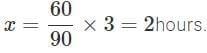QUESTION: 8

If 24 workers can paint a wall in 45 hours, how many workers are required to do the same work in 20 hours?

Solution:
QUESTION: 9

If the weight of 15 sheets of thick paper is 50 grams, how many sheets of the same paper would weigh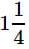kilograms?

Solution:
QUESTION: 10

If 32 horses consume 112 kg of gram in a certain period, how much gram will be consumed by 16 horses during the same period?

Solution:
QUESTION: 11

x varies inversely as square of y. Given that y = 3 for x = 1.find the value of x for y = 4.

Solution:
QUESTION: 12

The price of 357 mangoes is Rs.1517.25. What will be the approximate price of 49 dozens of such mangoes?

Solution:

Price of 1 mango = 1517.25/357

Price of 49 dozen of mangoes = 1517.25 x 49 x 12 / 357

= 2498.588 ≈ Rs 2500

QUESTION: 13

A contractor can complete a certain piece w of work in 9 days. He employed certain number of men, but 6 of them being absent from the very first day, the rest could finish the work in 15 days. How many men were originally employed?

Solution:
QUESTION: 14

If 11.25 m of a uniform iron rod weighs 42.75 kg,what will be the weight of 6 mof the same rod?

Solution:

Let the required weight be x kg. The length and weight are in direct proportion.

∴ 11.25:6::42.75 : x ⇒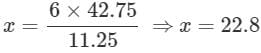QUESTION: 15

On a scale of a map, 0.6 cm represents 6.6 km If the distance between the points on the map is80.5 cm. What is the actual distance between these places?

Solution:
QUESTION: 16

A flagstaff 17.5 m high casts a shadow of length40.25 m. What is the height of the building which casts a shadow of length 28.75 m under similar conditions?

Solution:
QUESTION: 17

36 men can construct a bridge in 18 days. In how many days will 27 men complete the construction?

Solution:
QUESTION: 18

A rope makes 70 rounds of the circumference of a cylinder whose radius of the base is14 cm How many times can it go round a cylinder with radius 20 cm?

Solution:
QUESTION: 19

If a quarter kg of green chillies cost 60 paise, how many paise will 200 g cost?

Solution:

Let the required cost be x paise. Cost and weight are in direct proportion.

∴ 250 : 200 :: 60 : x

⇒ 250 × x = 200 × 60

⇒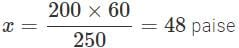QUESTION: 20

A recipe for 20 rolls needs 5 tablespoons w of butter. How many tablespoons of butter is needed for 30 rolls?

Solution:Use Code STAYHOME200 and get INR 200 additional OFF Use Coupon Code